UIHistories Project: A History of the University of Illinois by Kalev Leetaru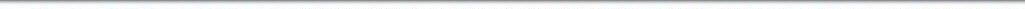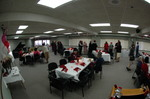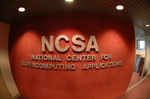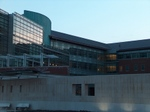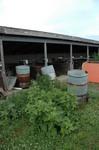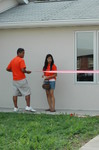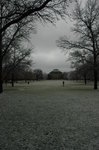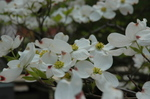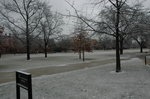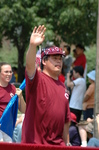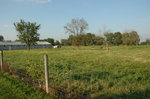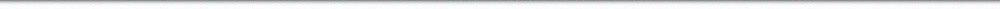N A V I G A T I O N D I G I T A L L I B R A R Y

## Repository: UIHistories Project: Board of Trustees Minutes - 1896 [PAGE 58]

Caption: Board of Trustees Minutes - 1896
This is a reduced-resolution page image for fast online browsing.

< Previous Page [Displaying Page 58 of 371] Next Page >
[VIEW ALL PAGE THUMBNAILS]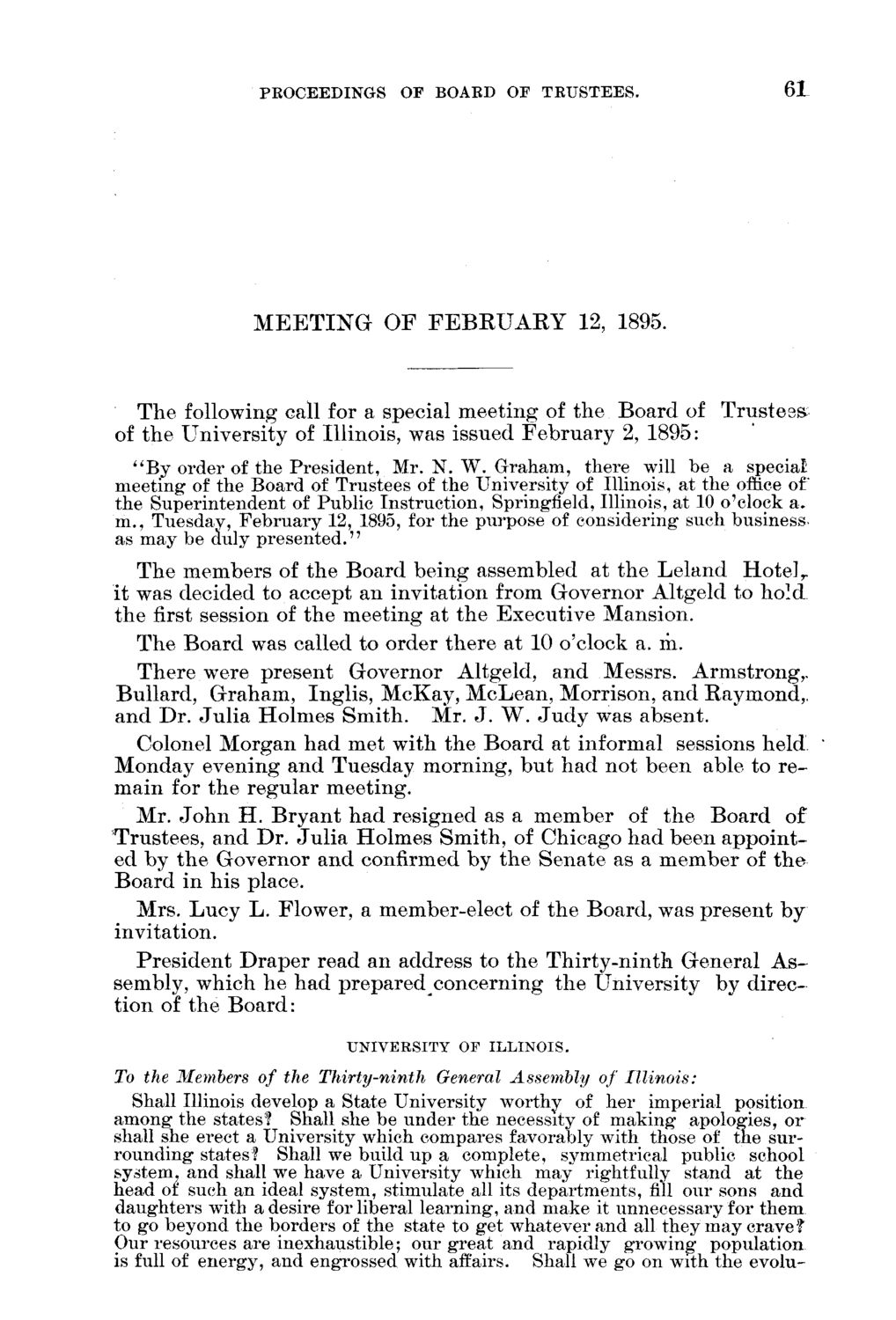### EXTRACTED TEXT FROM PAGE:

PROCEEDINGS OF BOARD OF TRUSTEES.

61

M E E T I N G O F F E B R U A R Y 12, 1895.

T h e following call for a special m e e t i n g of t h e B o a r d of of t h e U n i v e r s i t y of Illinois, was issued F e b r u a r y 2, 1895:

Trustees

"By order of the President, Mr. N. W. Graham, there will be a special meeting of the Board of Trustees of the University of Illinois, at the office of the Superintendent of Public Instruction, Springfield, Illinois, at 10 o'clock a. m., Tuesday, February 12, 1895, for the purpose of considering such business as may be duly presented." T h e m e m b e r s of t h e B o a r d b e i n g assembled at t h e L e l a n d H o t e l T it was decided to accept an i n v i t a t i o n from G o v e r n o r A l t g e l d to h o l d t h e first session of t h e m e e t i n g at t h e E x e c u t i v e M a n s i o n . T h e B o a r d was called to order t h e r e at 10 o'clock a. rii. T h e r e were p r e s e n t G o v e r n o r Altgeld, a n d Messrs. Armstrong,. B u l l a r d , G r a h a m , I n g l i s , M c K a y , M c L e a n , Morrison, a n d Raymond,, a n d D r . J u l i a H o l m e s S m i t h . Mr. J . W . J u d y was absent. Colonel M o r g a n h a d m e t with t h e B o a r d at informal sessions h e l d M o n d a y evening a n d Tuesday m o r n i n g , b u t h a d n o t been able to r e m a i n for t h e r e g u l a r m e e t i n g . Mr. J o h n H . B r y a n t h a d resigned as a m e m b e r of t h e B o a r d of T r u s t e e s , a n d D r . J u l i a H o l m e s S m i t h , of Chicago h a d been a p p o i n t ed by t h e G o v e r n o r a n d confirmed by t h e S e n a t e as a m e m b e r of t h e B o a r d in his place. M r s . L u c y L . Flower, a member-elect of t h e Board, was p r e s e n t b y invitation. P r e s i d e n t D r a p e r read an address to t h e T h i r t y - n i n t h G e n e r a l A s sembly, which h e h a d p r e p a r e d c o n c e r n i n g t h e U n i v e r s i t y by d i r e c tion of t h e B o a r d :

UNIVERSITY OF ILLINOIS.

To the Members of the Thirty-ninth General Assembly of Illinois: Shall Illinois develop a State University worthy of her imperial position among the states? Shall she be under the necessity of making apologies, or shall she erect a University which compares favorably with those of the surrounding states? Shall we build up a complete, symmetrical public school system, and shall we have a University which may rightfully stand at the head of such an ideal system, stimulate all its departments, fill our sons and daughters with a desire for liberal learning, and make it unnecessary for them to go beyond the borders of the state to get whatever and all they may crave? Our resources are inexhaustible; our great and rapidly growing" population is full of energy, and engrossed with affairs. Shall we go on with the evolu-